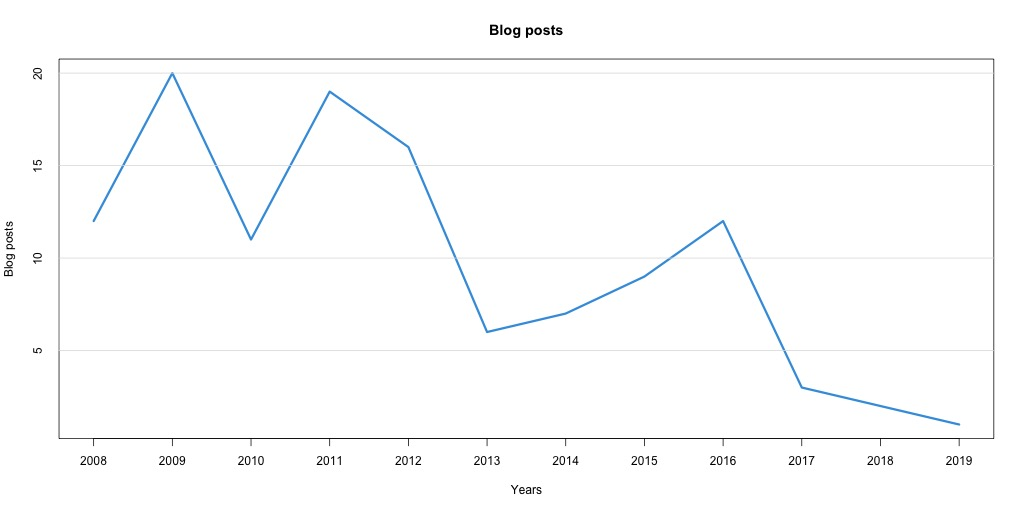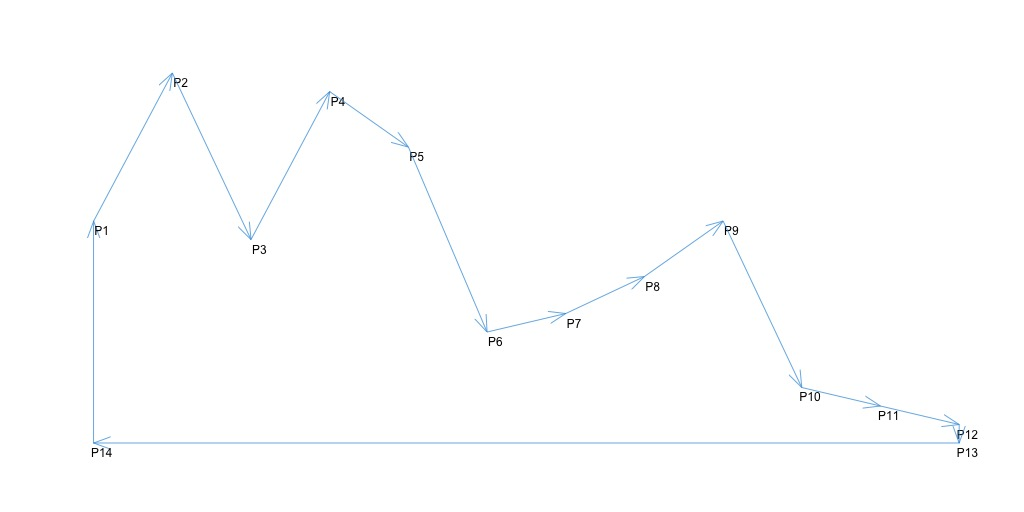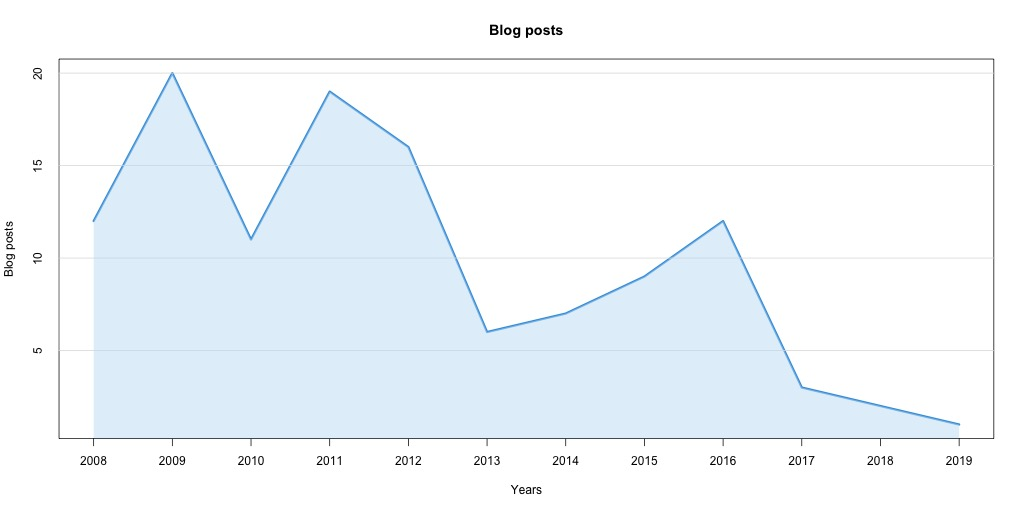# Area Charts using R base Graphics

Today I learnt to create Area chart using R Base graphics. polygon function (help(polygon)) can be used to fill the area under the line.

Lets pick a simple data set from my website - Number of blog posts per year.

```Year,Blog
2008,12
2009,20
2010,11
2011,19
2012,16
2013, 6
2014, 7
2015, 9
2016,12
2017, 3
2018, 2
2019, 1
```

Now lets draw the line graph as below.

```dat <- read.csv("blogs_data.csv")
numYears <- length(dat\$Blog)

plot(
c(1:numYears),     # x axis
dat\$Blog,          # y axis
type="l",          # Line graph
col="#409ddf",     # Line Color
lwd=3,             # Line Width
xlab="Years",      # X axis label
ylab="Blog posts", # Y axis label
xaxt="n",          # Show X axis
yaxt="n",          # Show Y axis
main="Blog posts", # Graph main title
)

axis(1, at=1:numYears, labels=sprintf("%s", dat\$Year))
axis(2, tck=1, col="#dddddd")
```Identify the points required to draw a polygon as below,```yy <- c(0, dat\$Blog, 0, 0)          # P14, P1:P12, P13, P14
xx <- c(1, 1:numYears, numYears, 1) # P14, P1:P12, P13, P14
```

And draw polygon using,

```polygon(xx, yy, col="#c3e1f57d", border=NA)
```

And the final Area chart,